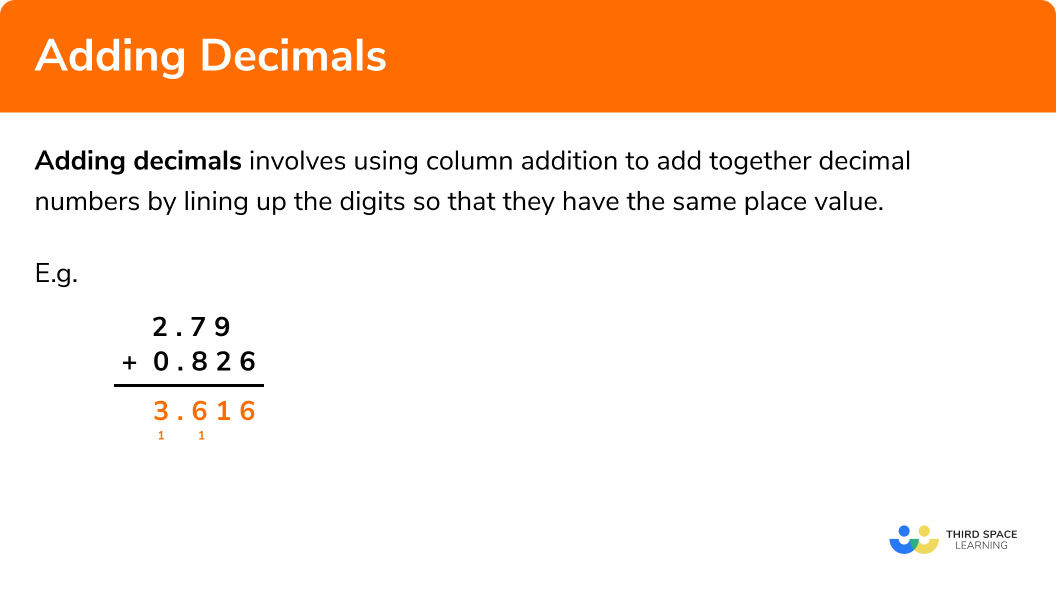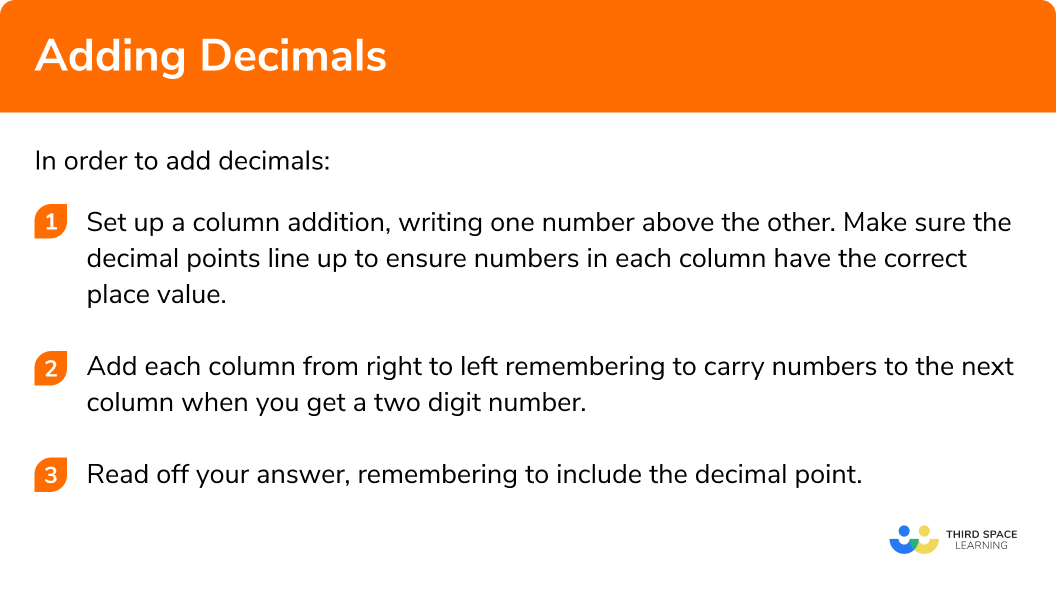There are also adding decimals worksheets with word problems and problem solving questions based on Edexcel, AQA and OCR exam questions, along with further guidance on where to go next if you’re still stuck.

Adding decimals involves using column addition to add together decimal numbers by lining up the digits so that they have the same place value.

The ones, decimal points, tenths, hundredths etc. should be lined up. This is particularly important to do if the values we are adding have been given to a different number of decimal places.

E.g.

A similar approach will be used for decimal subtraction.1. Set up a column addition, writing one number above the other. Make sure the decimal points line up to ensure numbers in each column have the correct place value.
2. Add each column from right to left remembering to carry numbers to the next column when you get a two digit number.

### Explain how to add decimals in 3 steps### Related lessons on decimals

Adding decimals is part of our series of lessons to support revision on decimals. You may find it helpful to start with the main decimals lesson for a summary of what to expect, or use the step by step guides below for further detail on individual topics. Other lessons in this series include:

### Example 1: adding numbers with one decimal place

1. Set up a column addition, writing one number above the other. Make sure the decimal points line up to ensure numbers in each column have the correct place value.

2Add each column from right to left remembering to carry numbers to the next column when you get a two digit number.

### Example 2: adding numbers with two decimal places

Find the sum of 9.18 and 7.35.

### Example 3: adding numbers with three decimals places

Work out 4.275 + 12.836.

### Example 4: adding whole numbers and decimal numbers

Fill in missing zeros if it helps.

### Example 5: adding numbers with different amounts of decimal places

Find the sum of 6.4 and 13.701.

Fill in missing zeros if it helps.

### Example 6: adding more than two decimal numbers

Work out 3.79 + 2.184 + 14.6.

Fill in missing zeros if it helps.

### Common misconceptions

• Not lining up the decimal point

A common error when adding decimals occurs when the decimal points are not lined up but instead the last digits are lined up.

E.g.

1. Add together 3.7 and 5.6

9.38.138.39.13You must remember to line up the decimal points carefully and carry to the next column if the sum of the digits gives a two digit answer.2. Work out £12.84 + £6.19

£18.103£19.03£18.913£19.3You must remember to line up the decimal points carefully and carry to the next column if the sum of the digits gives a two digit answer.3. Find the sum of 0.069 and 5.987

5.10566.6776.566.056You must remember to line up the decimal points carefully and carry to the next column if the sum of the digits gives a two digit answer.4. Add together 2 and 3.87

5.873.894.0723.87You must remember to line up the decimal points carefully, you can fill in missing zeros if it helps.5. Find the sum of 9.04 and 17.381

26.42118.285107.78126.385You must remember to line up the decimal points carefully and carry to the next column if the sum of the digits gives a two digit answer. You can fill in missing zeros if it helps.6. Work out 20.3 + 13.67 + 0.998

25684.39534.96834.068You must remember to line up the decimal points carefully and carry to the next column if the sum of the digits gives a two digit answer. You can fill in missing zeros if it helps.1.

(a) Work out £ 13.67 + £ 8.59

(b) Work out 55.6 + 2.392 + 12.48

(3 marks)

a)

(1)

b)

57.992 or 68.08 or 14.872 seen

(1)

(1)

2. Complete the following calculations

(a)(b)(5 marks)

a)

2 in first box

(1)

7 in second box(1)

b)

8 in top box

(1)

1 in first box in second row

(1)

6 in second box in second row(1)

3. Find the perimeter of the irregular pentagon.(2 marks)

4.1+3.5+2.9+4.6+3.8

(1)

(1)

## Learning checklist

You have now learned how to:

• Understand and use place value for decimals, measures and integers of any size
• Use the four operations, including formal written methods, applied to integers, decimals, proper and improper fractions, and mixed numbers, all both positive and negative

## Still stuck?

Prepare your KS4 students for maths GCSEs success with Third Space Learning. Weekly online one to one GCSE maths revision lessons delivered by expert maths tutors.

Find out more about our GCSE maths tuition programme.